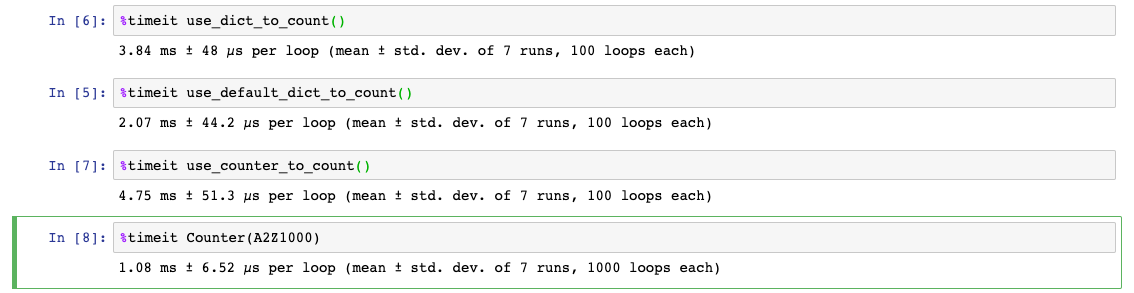# Designed for Counting - Python Counter Class

Posted on  Apr 19, 2022  in  Python Programming - Beginner Level  by  Amo Chen  ‐ 5 min read

The Python collections module provides several convenient classes for developers to use, among which the Counter class (a subclass of dict) can be applied in counting-related scenarios:

A Counter is a dict subclass for counting hashable objects.

This article will introduce the usage of the Counter class and compare its performance with dict and defaultdict.

• Python 3.7

### An introduction to the Counter class #

Counting for general statistical items, we usually use dict (also called dictionary) intuitively, for example, the following use dict to count the number of words, stopwords, and sentences:

``````counters = {
"words": 123,
"stopwords": 4,
"sentences": 6,
}
``````

When using dict for statistics, it is inevitable to judge whether the key already exists in the dictionary. If it does not exist, assign a default value. If it already exists, add or subtract the count according to the requirements, such as the following code:

``````if key in counters:
counters[key] += 1
else:
counters[key] = 1
``````

In addition to the above mentioned methods for checking if the key exists, setdefault() can also be used to set a default value for a non-existent key, as the following example:

``````counters.setdefault(key, 0)
counters[key] += 1
``````

Counter is a new class added to Python after version 3.1, which can be said to have integrated the aforementioned practices and further simplified them. After using Counter, developers no longer need to check whether a key exists in the dictionary, nor do they need to set a default value for the key (it defaults to 0), so the usage can be directly simplified to:

``````from collections import Counter

counters = Counter(
{
'words': 123,
'stopwords': 4,
'sentences': 6,
}
)

counters['words'] += 1
counters['new item'] += 1
``````

Also, because Counter inherits from dict, it can also be traversed in the same way as dict, for example:

``````from collections import Counter

counters = Counter(
{
'words': 123,
'stopwords': 4,
'sentences': 6,
}
)

for k, v in counters.items():
print(k, v)
``````

The built-in methods of the Counter class are also useful, such as the most_common() which can help us get the top n key and value and sort them in descending order, for example, to get the top 3 key and value in counters as follows.

``````>>> counters.most_common(3)  # TOP 3
[('words', 123), ('sentences', 6), ('stopwords', 4)]
``````

The Counter class accepts arguments as initial values, which can be iterable, dict, or even keyword arguments, as shown in the following example, where all results are the same.

``````counters = Counters({'a': 1, 'b': 2})

counters = Counter(['a', 'b', 'b'])

counters = Counters('abb')

counters = Counters(a=1, b=2)
``````

Counter also supports `+` `-` operations, where addition combines all keys from two Counters and sums up values for equal keys:

``````>>> Counter(a=1, b=1) + Counter(a=1, b=1, c=1)
Counter({'a': 2, 'b': 2, 'c': 1})
``````

Subtraction operation only subtracts the same key values, and if the value is 0 after subtraction, the key value is deleted.

``````>>> Counter(a=3, b=1) - Counter(a=1, b=1, c=1)
Counter({'a': 2})
``````

In addition, Counter also supports `|` (Bitwise Or) and `&` (Bitwise And) two operations, just like set().

Using `|` (Bitwise Or) will leave the maximum value of each key in the Counter:

``````>>> Counter('abbb') | Counter('bcc')
Counter({'b': 3, 'c': 2, 'a': 1})
``````

Using `&` (Bitwise And) will only keep the intersection of each key with the minimum value of all the Counters:

``````>>> Counter('abbbc') & Counter('bcc')
Counter({'b': 1, 'c': 1})
``````

### Performance Benchmarking #

At the last, let’s compare the execution efficiency of using dict, defaultdict, and Counter.

The following example programs use dict, defaultdict, and Counter respectively to calculate the number of occurrences of each character in the long string A2Z1000 (a to z repeated 1000 times), and use `%timeit` in Jupyter notebook to measure their execution time:

``````from collections import Counter, defaultdict

A2Z1000 = ''.join(chr(i) for i in range(97, 123)) * 1000

def use_dict_to_count():
d = dict()
for c in A2Z1000:
d.setdefault(c, 0)
d[c] += 1

def use_default_dict_to_count():
d = defaultdict(int)
for c in A2Z1000:
d[c] += 1

def use_counter_to_count():
d = Counter()
for c in A2Z1000:
d[c] += 1
``````The above results show that `use_counter_to_count()` is slower than using a dict or defaultdict alone.

However, interestingly, when we simply switched to using `Counter(A2Z1000)`, the efficiency actually became extremely good! Why is this the case?

Actually, when initializing a Counter instance with an iterable, Counter will call a helper function implemented in C - _count_elements. This function not only benefits from the efficiency of the C language, but also reduces many of the overheads of Python’s inherent object-oriented operations, which is why it is relatively faster.

If you want to improve the efficiency of Counter, it is recommended to use the `Counter(iterable)` method for calculation, and you can also call the [update()](https://docs.python.org/3/library/collections.html#collections.Counter.

To improve the efficiency of Counter, it is recommended to not only use `Counter(iterable)` to perform the calculation, but also to call the update() method provided by Counter (this method does not overwrite key values like dict and defaultdict, but adds them up). As long as iterable is passed in, this method will also call _count_elements for calculation. Therefore, `use_counter_to_count()` can be further modified as follows to be faster than dict and defaultdict:

``````def use_counter_to_count():
d = Counter()
d.update(A2Z1000)
``````

That’s all about the Introduction of the Counter class!

Happy Coding!

https://github.com/python/cpython/blob/7acedd71dec4e60400c865911e8961dbb49d5597/Lib/collections/init.py#L534

https://docs.python.org/3/library/collections.html#collections.Counter